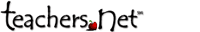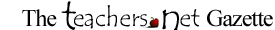chat centerSUBSCRIBEMY LINKS:The Gazette Chatboards Mailrings Classifieds Lessons Jobs Harry Wong Projects Chatroom Meetings AdvertiseTEACHERS.NET GAZETTE
SEPTEMBER 2000
Volume 1 Number 7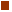COVER STORY Ride along with the Hole in the Wall Gang this month and discover the special camp founded by Paul Newman nestled away in the quiet hills of Connecticut.COLUMNS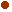Effective Teaching by Harry WongPromoting Learning by Marv MarshallThe Trouble With... by Alfie Kohn4 Blocks by Cheryl SigmonSchoolhouse Views by Beth BrunoARTICLES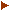To Refer or Not to ReferTell A Number TrickBCL Classroom EnvironmentsLinks Worth The ClickMorning MeetingsFLingers Block PartyBridging the Digital DivideScience Teacher InitiativePoetry Contest for Canadian YouthDeveloping a Positive Home-School RelationshipClassroom Rules Can Be SweetTeaching the Visually ImpairedBJ Treks OutbackTeacher To Ski AntarcticaREGULAR FEATURES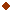New at Teachers.NetLetters to the EditorPoll: Favorite QuotesArchives: Self PublishingNew in the Lesson BankUpcoming Ed ConferencesHumor from the ClassroomHelp Wanted - Teaching JobsLive Events CalendarGazette Back IssuesGazette Home Delivery:

Mr. Emekwulu is originally from Nigeria. Before he immigrated to the United States, he taught mathematics at Boys� High School, Uga in Anambra State of Nigeria and also at St. Mark�s Teachers Training College, Nibo-Nise also in Anambra state. Before teaching at Uga Boys�, he had taught at Central and Community Primary Schools, Ebenato in Nnewi local government area of Anambra state, Nigeria. About his Works He has been inspiring his audiences since 1992 with his seminar series - Can I publish my own book?, Yes, There Could Be A Book In You and Math-Magic, a creative and innovative way of motivating high school students in appreciating mathematics. An award - winning author, Emekwulu is the author of Magic of Numbers, Grades 9-12, Fibonacci Numbers For Research Mathematicians and AI Applications. His several unpublished manuscripts include Beauty of Mathematics, Vols. 1 & 2, Mathematical Encounters, Awakening Your Writing Genius etc. Emekwulu�s primary interest lies in exploring properties of Fibonacci and triangular numbers. He has organized seminars for National Council of Teachers of Mathematics and other professional organizations including Moore Association of Classroom Teachers, Oklahoma State Education Association, Liberated Arts Center in Oklahoma, City, Jenks Public Schools, Oklahoma State University, Stillwater, University of Oklahoma, Board Members of Organization of Rural Oklahoma Schools and several high schools, Oklahoma City Writers, Norman Galaxy of Writers, Mid-Oklahoma Writers Club etc. He has also presented an online self-publishing seminar for Teachers.Net.

Paul is a member of Association of Supervision and Curriculum Development (ASCD). He is available for staff development and classroom presentation of Math-Magic. He can be contacted at 405.447.9019 or novelty@telepath.com or P. O. Box 2482, Norman, OK 73070. Information about his books, seminars and others can be found by visiting his web site at www.telepath com/novelty or at www.amazon.com or www.barnesandnoble.com

Best Sellers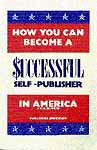How You Can Become a Successful Self-Publisher in America and Elsewhere
by Paul Chika Emekwulu

\$19.95 from Amazon.com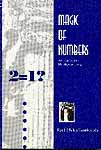Magic of Numbers
by Paul Chika Emekwulu

\$19.95 from Amazon.com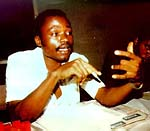Tell A Number Trick
by Paul Chika Emekwulu, Author - "Magic of Numbers"

"Let's play a game," Lori said to her friend, Tessi. Both are students at Bahome High School, North - East Potomico.
"What kind of game is that?" Tessi asked in return.
"What you need is just a piece of paper and a pencil," Lori continued.

Lori and Tessi have started the game. Below are the instructions:

1. Choose any number with two or more digits, e.g. 467896
2. Add the digits, i.e. ( 4 + 6 + 7 + 8 + 9 + 6 ) = 40
3. Subtract this sum from the original number, i.e.467896 - 40 = 467856
4. Cross out any digit you like and read out the remaining digits in order: 4 -6 - 7 - 8 -5 (gradually).

Now, how do we know the number Tessi crossed out? Do you want to know? Now listen and make sure you follow the investigation carefully. Promise to go diligently with me through this investigation and find out the number Tessi crossed out.

### Now, the algebraic investigation

1. Let's take a number, say 54. By adding the digits we have: ( 5 + 4 ) = 9
2. Subtract this sum from the original number, i.e. 54 - 9 = 45
Let's take more numbers, e.g. 74. Then 7 + 4 = 11 and 74 - 11 = 63.
Another number, say 87. 8 + 7 = 15 and 87 - 15 = 72
At this stage, it seems that the number left after subtraction is always divisible by 9. We don't know yet. It is only an assumption at this point. What do you think? If the above assumption is true, it means that such a number will beautifully obey the divisibility rule of 9.
Back to our example, 54.
3. Adding the digits in 54, we have 5 + 4 = 9.
4. Now, let's cross out any digit in 54. Which do you want crossed out? It doesn't make any difference. Crossing out 4, we are now left with 5.
At this point of the investigation, let's go back to the divisibility by 9 and get ourselves acquainted once more with it. This test states that a number is divisible by 9 if the sum of its digits is divisible by 9.
Do you still remember this rule?
5. In our example, since the number 5 left after crossing out 4 is only a one digit number, the number left after the procedural mental addition is 5. But let me ask you a question.
If in the number left and after subtracting the sum of digits, I cross out a digit, how else do you think we can easily find the number crossed out other than by applying the divisibility rule of 9? Think about this.
6. Now let us represent 4 in 54 with m. Since 54 is divisible by 9, then, 5 + m (sum of digits) is also divisible by 9. At this point, what do you think about the crossed out digit?
Now, listen. What number should be added to 5 such that the resulting number is divisible by 9? Think of 4, 13, 22, 31, 40... as possibilities.
Again, remember that the number crossed out is a one digit number. From the above series, what is the number satisfying our condition for the values which m can take?
Therefore, the smallest value m can take is 4 so that 5 + m will be divisible by 9.

How? From the above you will see that:

5 + 4 = 9
5 + 13 = 18
5 + 22 = 27
5 + 31 = 36
5 + 40 = 45

These sums are all multiples of 9. From the above, the highest multiple of 9 with respect to 5 is 9. Therefore, m (number crossed out) = 9 - 5 = 4.

Now, let's have a general look at the situation. Consider a number, abcd, whose other name is 1000a + 100b + 10c + d. This expression could be expressed further as:

( 999a + a ) + ( 99b + b ) + ( 9c + c ) + d

This last expression in turn, also is equal to:

( 999a + 99b + 9c ) + ( a + b + c + d ) = 9 ( 111a + 11b + c ) + ( a + b + c + d )

The investigation continues.

a + b + c + d represents the sum of digits in the original number, abcd. Therefore, subtracting this sum from the original number, which is equal to:

9 ( 111a + 11B + c ) + ( a + b + c + d )

we have,

9 ( 111a + 11b + c ) + ( a + b + c + d ) - a - b - c - d = 9 ( 111a + 11b + c )

The expression, 9 ( 111a + 11b + c ) is a multiple of 9.

Do you agree? In other words, generally, the number left after subtracting the sum of digits from the original number, abcd, is always a multiple of 9. It is therefore, divisible by 9 since it has 9 as a factor.

Again, since 9 ( 111a + 11b + c ) = 1000a + 100b + 10c + d - ( a + b + c + d ), 1000a + 100b + 10c + d - ( a + b + c + d ) is, consequently, a multiple of 9.

Again, 1000a + 100b + 10c + d - ( a + b + c + d ) = 1000a + 100b + 10c + ( d - d ) - ( a + b + c ) = 1000a + 100b + 10c - ( p + q + r ) where p, q, r are the numbers left after the crossing out process.

When the original number is abcd, the numbers left after crossing out will be p, q, and r.
Do you agree with this logic?

Continuing our investigation, let 9k be a multiple of 9 where k is determined by the quantity,

p + q + r

Therefore, the number crossed out is the expression, 9k - ( P + q + r ).

1. Let's make an illustration with this example by choosing the number, 6345 as our original number.
2. Adding the digits we have: 6 + 3 + 4 + 5 = 18
3. Subtracting the sum of difits from the original number we have: 6345 - 18 = 6327
4. Let's cross out 6.
Then, we have 327 left.
Therefore, p = 3 , q = 2 , and r = 7
5. Adding p, q, and r, we have: 3 + 2 + 7 = 12
6. The next highest multiple of 9 more than 12 is 18. i.e. k = 2
7. Therefore, number crossed out = 9k - ( p + q + r ).
8. By substitution, m = 18 - ( 3 + 2 + 7 ) = 18 - 12 = 6

Experience has shown that students enjoy this trick. At all of my presentations, they always get excited and are always very anxious to understand the algebra explaining the trick.

An adaptation from Magic of Numbers, Grades 9-12 by Paul Chika Emekwulu

Magic of Numbers, Grades 9-12 is available at the following: amazon.com, barnesandnoble.com, borders.com or through the publisher:

Novelty Books
P.O.Box 2482
Norman, OK 73070
Tel/Phone: (405) 447 - 9019